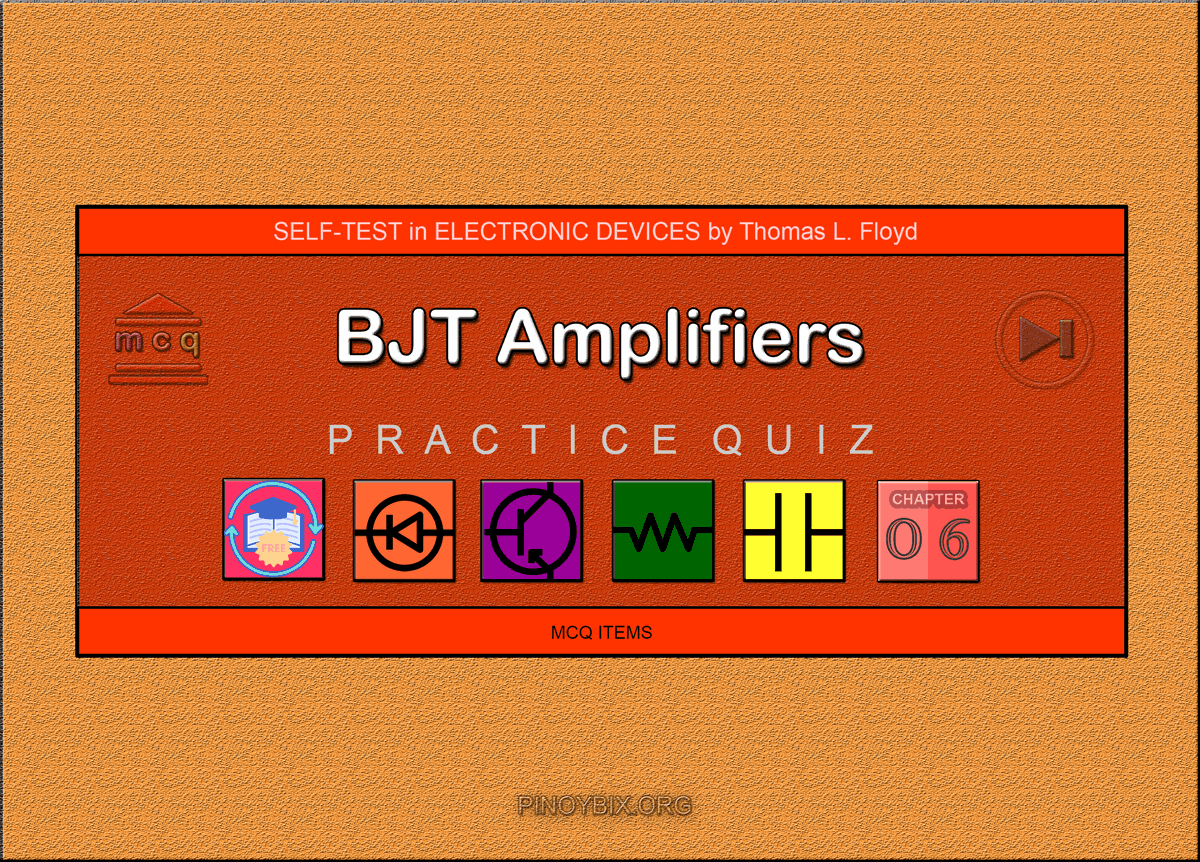# Floyd Self-test in BJT Amplifiers

(Last Updated On: December 5, 2019)This is the Self-test in Chapter 6: Bipolar Junction Transistor Amplifiers from the book Electronic Devices Conventional Current Version, 9th edition by Thomas L. Floyd. If you are looking for a reviewer in Electronics Engineering this will definitely help you before taking the Board Exam.

#### Floyd Self-test Chapter 6 Topic Outline

• Floyd Self-test in Bipolar Junction Transistors Amplifiers
• Floyd Self-test in Amplifier Operation
• Floyd Self-test in Transistor AC Models
• Floyd Self-test in The Common-Emitter Amplifier
• Floyd Self-test in The Common-Collector Amplifier
• Floyd Self-test in The Common-Base Amplifier
• Floyd Self-test in Multistage Amplifiers
• Floyd Self-test in The Differential Amplifier

If you are looking for the Multiple Choice Questions in Floyd’s Electronic Devices proceed to

#### Start Practice Exam Test Questions

Choose the letter of the best answer in each questions.

1. A small-signal amplifier

(a) uses only a small portion of its load line

(b) always has an output signal in the mV range

(c) goes into saturation once on each input cycle

(d) is always a common-emitter amplifier

Solution:

2. The parameter hfe corresponds to

(a) βdc

(b) βac

(c) r’e

(d) r’c

Solution:

3. If the dc emitter current in a certain transistor amplifier is 3 mA, the approximate value of is

(a) 3kΩ

(b) 3Ω

(c) 8.33Ω

(d) 0.33Ω

Solution:

4. A certain common-emitter amplifier has a voltage gain of 100. If the emitter bypass capacitor is removed,

(a) the circuit will become unstable

(b) the voltage gain will decrease

(c) the voltage gain will increase

(d) the Q-point will shift

Solution:

5. For a common-emitter amplifier, RC = 1.0 kΩ, RE = 390Ω, r e = 15 Ω, and = 75.

Assuming that RE is completely bypassed at the operating frequency, the voltage gain is

(a) 66.7

(b) 2.56

(c) 2.47

(d) 75

Solution:

6. In the circuit of Question 5, if the frequency is reduced to the point where XC (bypass)= RE, the voltage gain

(a)remains the same

(b) is less

(c) is greater

Solution:

7. In a common-emitter amplifier with voltage-divider bias, RIN(BASE)=68kΩ, R1= 33kΩ, and R2= 15kΩ. The total ac input resistance is

(a) 68kΩ

(b) 8.95kΩ

(c) 22.2kΩ

(d) 12.3kΩ

Solution:

8. A CE amplifier is driving a load. If the voltage gain is approximately

(a) 220

(b) 1000

(c) 10

(d) 180

Solution:

9. For a common-collector amplifier, RE= 100Ω, r’e =10Ω, and = 150. The ac input

resistance at the base is

(a) 1500 Ω

(b) 15k Ω

(c) 110 Ω

(d) 16.5 kΩ

Solution:

10. If a 10 mV signal is applied to the base of the emitter-follower circuit in Question 9, the output signal is approximately

(a) 100 mV

(b) 150 mV

(c) 1.5 V

(d) 10 mV

Solution:

11. In a certain emitter-follower circuit, the current gain is 50. The power gain is approximately

(a) 50AV

(b) 50

(c) 1

Solution:

12. In a Darlington pair configuration, each transistor has an ac beta of 125. If RE is the

input resistance is

(a) 560 Ω

(b) 70 kΩ

(c) 8.75M Ω

(d) 140 kΩ

Solution:

13. The input resistance of a common-base amplifier is

(a) very low

(b) very high

(c) the same as a CE

(d) the same as a CC

Solution:

14. Each stage of a four-stage amplifier has a voltage gain of 15. The overall voltage gain is

(a) 60

(b) 15

(c) 50,625

(d) 3078

Solution:

15. The overall gain found in Question 14 can be expressed in decibels as

(a) 94.1 dB

(b) 47.0 dB

(c) 35.6 dB

(d) 69.8 dB

Solution:

16. A differential amplifier

(a) is used in op-amps

(b) has one input and one output

(c) has two outputs

Solution:

17. When a differential amplifier is operated single-ended,

(a) the output is grounded

(b) one input is grounded and a signal is applied to the other

(c) both inputs are connected together

(d) the output is not inverted

Solution:

18. In the double-ended differential mode,

(a) opposite polarity signals are applied to the inputs

(b) the gain is 1

(c) the outputs are different amplitudes

(d) only one supply voltage is used

Solution:

19. In the common mode,

(a) both inputs are grounded

(b) the outputs are connected together

(c) an identical signal appears on both inputs

(d) the output signals are in-phase

Solution:

### Complete List of Floyd Self-test in Electronic Devices

P inoyBIX educates thousands of reviewers and students a day in preparation for their board examinations. Also provides professionals with materials for their lectures and practice exams. Help me go forward with the same spirit.

“Will you subscribe today via YOUTUBE?”

Subscribe

PinoyBIX Engineering. © 2014-2020 All Rights Reserved | How to Donate? |